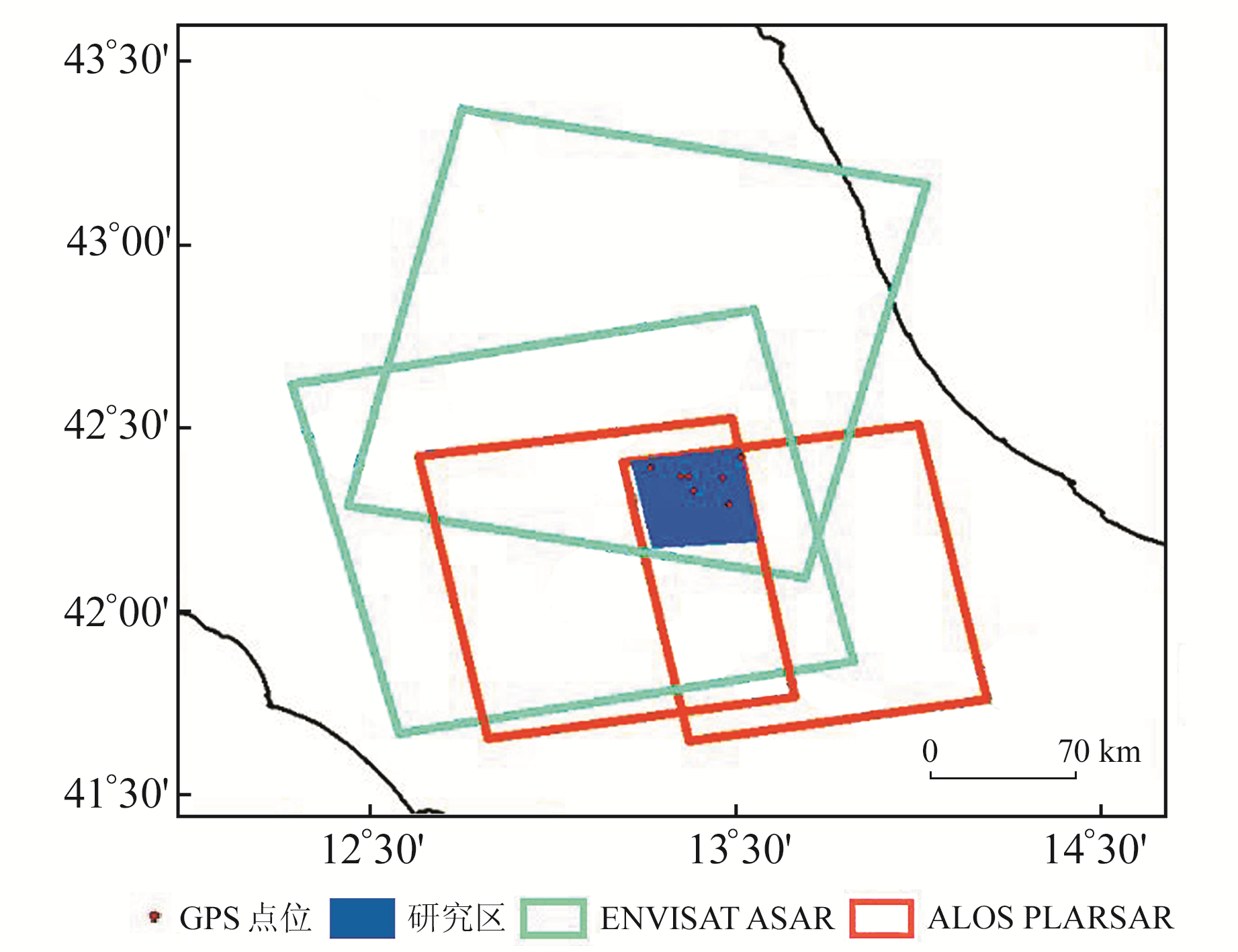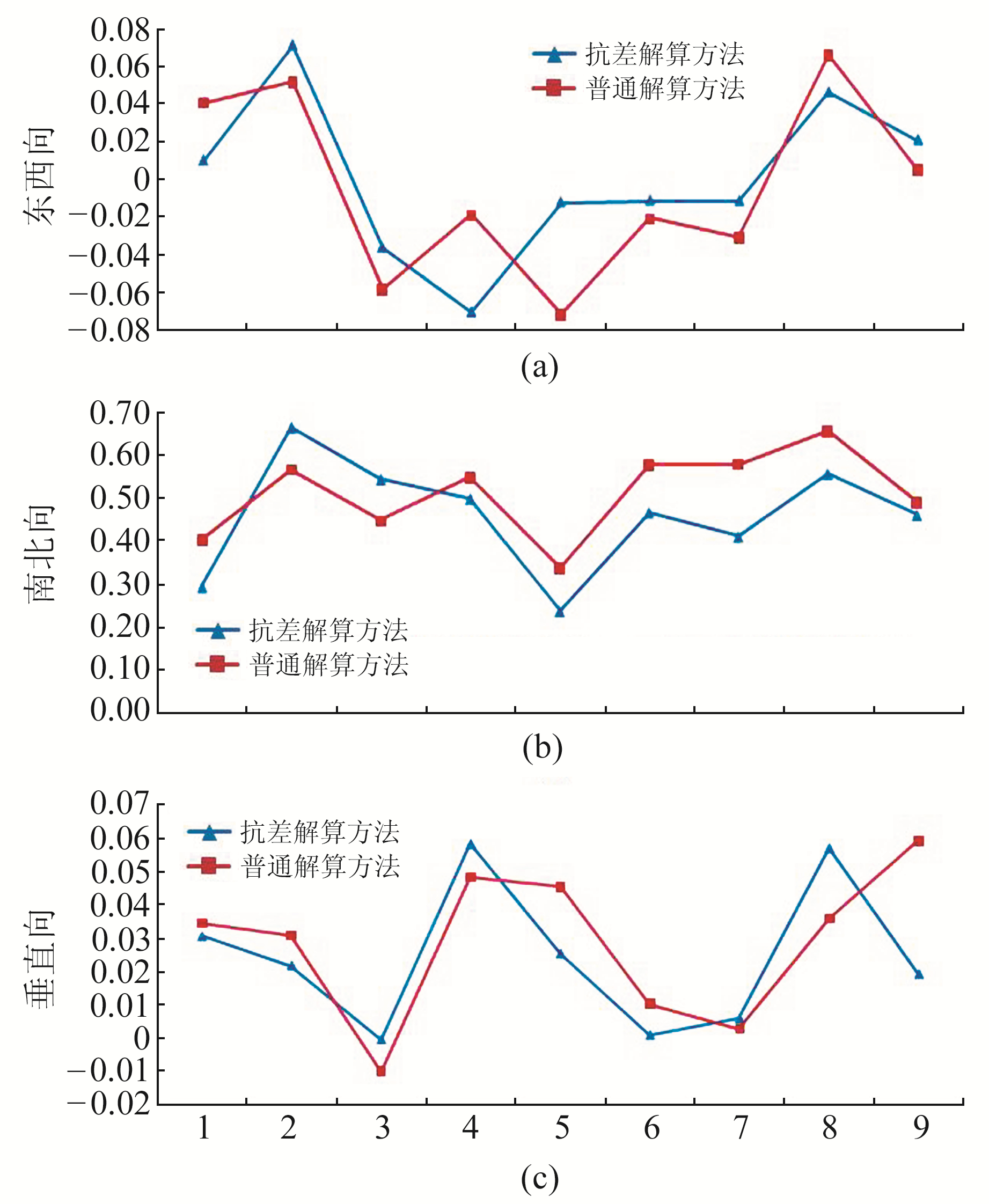﻿ 基于多视线向D-InSAR技术的三维地表形变抗差解算方法文章快速检索 高级检索
 大地测量与地球动力学2020, Vol. 40Issue (12): 1263-1267  DOI: 10.14075/j.jgg.2020.12.011### 引用本文ZHOU Lixin, WANG Zhiwei. Robust Method for Resolving 3-D Surface Deformation from Multi-LOS D-InSAR Technology[J]. Journal of Geodesy and Geodynamics, 2020, 40(12): 1263-1267.### Foundation support

PhD Scientific Research Foundation of Shandong Jianzhu University, No.X18097Z.

### Corresponding author

WANG Zhiwei, PhD, lecturer, majors in InSAR 3-D surface deformation calculating, E-mail:zhi.wei.wang@163.com.

### About the first author

ZHOU Lixin, senior engineer, majors in modern measurement data processing, E-mail:54775725@qq.com.

### 文章历史

1. 山东省物化探勘查院, 济南市历山路56号, 250014;
2. 山东建筑大学测绘地理信息学院, 济南市凤鸣路1000号, 250101

1 多视线向D-InSAR三维地表形变抗差解算方法

$\mathit{\boldsymbol{d}} = {\left( {{d_{\rm{U}}}, {d_{\rm{E}}}, {d_{\rm{N}}}} \right)^{\rm{T}}}$为三维地表形变信息，$\mathit{\boldsymbol{R = }}{\left[ {R_{{\rm{LOS}}}^1, R_{{\rm{LOS}}}^2, \cdots , R_{{\rm{LOS}}}^n} \right]^{\rm{T}}}$为雷达坐标系中不同视线向形变信息，建立如下形变模型：

 $\mathit{\boldsymbol{R}} = \mathit{\boldsymbol{Bd}}$ (1)

 $\mathit{\boldsymbol{d}} = {\left( {{\mathit{\boldsymbol{B}}^{\rm{T}}}\mathit{\boldsymbol{PB}}} \right)^{ - 1}}{\mathit{\boldsymbol{B}}^{\rm{T}}}\mathit{\boldsymbol{PR}}$ (2)

 $\left\{ \begin{array}{l} \sigma _{0i}^2 = \mathit{\boldsymbol{V}}_i^T{\mathit{\boldsymbol{P}}_i}{\mathit{\boldsymbol{V}}_i}/{z_i}\\ {z_i} = {n_i} - {\rm{tr}}\left( {{\mathit{\boldsymbol{P}}_i}{\mathit{\boldsymbol{N}}_i}{\mathit{\boldsymbol{N}}^{ - 1}}\mathit{\boldsymbol{N}}_i^T} \right)\\ \mathit{\boldsymbol{N}} = {\mathit{\boldsymbol{B}}^T}\mathit{\boldsymbol{PB}}, {\mathit{\boldsymbol{N}}_i} = \mathit{\boldsymbol{B}}_i^T{\mathit{\boldsymbol{P}}_i}{\mathit{\boldsymbol{B}}_i}, i = 1, 2 \end{array} \right.$ (4)

 $\mathop \sum \limits_{i = 1}^r \left( {\mathit{\boldsymbol{B}}_i^T{{\mathit{\boldsymbol{\bar P}}}_i}{\mathit{\boldsymbol{B}}_i}\mathit{\boldsymbol{d}} - \mathit{\boldsymbol{B}}_i^T{{\mathit{\boldsymbol{\bar P}}}_i}{\mathit{\boldsymbol{R}}_i}} \right) = 0$ (5)

 $\mathit{\boldsymbol{\bar P}} = {\mathit{\boldsymbol{P}}_1}, \mathit{\boldsymbol{\bar P}} = \frac{{\sigma _{01}^2}}{{\sigma _{02}^2}}{\mathit{\boldsymbol{P}}_2}$ (6)

2 拉奎拉地震三维地表形变解算及结果分析 2.1 实验区与数据源

2009-04-06意大利拉奎拉市发生MW6.3地震，地震发生后，欧空局公布了ENVISAT ASAR数据以及日本的ALOS PALSAR数据，包括地震前和地震后升、降轨数据等。本文以该地震震中区域为研究区来验证所提出三维地表形变抗差解算方法的有效性，所选取的4个干涉像对SAR影像覆盖范围如图 1所示，基本参数如表 1所示。图 1 研究区概况 Fig. 1 Overview of the research area表 1 SAR影像基本参数 Tab. 1 Basic imaging parameters of SAR
2.2 数据处理图 2 拉奎拉地震差分干涉相位图 Fig. 2 Interferogram of L'Aquila earthquake

2.3 三维地表形变解算图 3 三维地表形变解算结果 Fig. 3 Results of three-dimensional surface deformation
2.4 精度验证图 4 三维地表形变结果与真实GPS数据结果的对比 Fig. 4 Comparison of resolved three-dimensional deformation and GPS results表 2 对比结果 Tab. 2 Comparison results
3 结语

1) 2种传感器SAR影像都获取了具有明显干涉条纹的差分干涉图并且干涉条纹位置基本一致，但干涉条纹在具体覆盖区域大小以及疏密程度等方面略有不同。采用不同传感器SAR影像进行地表形变监测可以获取更丰富的地表形变监测结果。

2) 采用多视线向D-InSAR三维地表形变抗差解算方法可以有效获取研究区内三维地表形变场。从形变分布图中可以明显看出本次地震断裂带所在的位置，为研究地震断层滑动分布提供更直接的地表形变监测结果。

3) 相对于普通加权最小二乘获取的三维地表形变解算结果而言，本文所提出的抗差最小二乘解算方法在3个方向上的精度都有所改善，证明了其有效性。

  Hanssen R F. Radar Interferometry Data Interpretation and Error Analysis[J]. Journal of the Graduate School of the Chinese Academy of Sciences, 2001, 2(1): 575-580 (0)  刘国林, 赵长胜, 张书毕, 等. 近代测量平差理论与方法[M]. 徐州: 中国矿业大学出版社, 2012 (Liu Guolin, Zhao Changsheng, Zhang Shubi, et al. The Theory and Method of Modern Surveying Adjustment[M]. Xuzhou: China University of Mining and Technology Press, 2012) (0)  康开轩, 邢灿飞, 李辉, 等. 抗差Helmert方差分量估计在重力网平差中的应用[J]. 大地测量与地球动力学, 2008, 28(5): 115-119 (Kang Kaixuan, Xing Canfei, Li Hui, et al. Application of Robust Helmert Method of Variance Component Estimation in Adjustment of Gravity Network[J]. Journal of Geodesy and Geodynamics, 2008, 28(5): 115-119) (0)  Yang H L, Peng J H. Mapping Three-Dimensional Co-Seismic Deformations Fields by Combining Multiple-Aperture Interferometry and Differential Interferometric Synthetic Aperture Radar Techniques[J]. Journal of the Indian Society of Remote Sensing, 2016, 44(2): 243-251 DOI:10.1007/s12524-015-0484-y (0)  杨元喜, 宋力杰, 徐天河. 大地测量相关观测抗差估计理论[J]. 测绘学报, 2002, 31(2): 95-99 (Yang Yuanxi, Song Lijie, Xu Tianhe. Robust Parameter Estimation for Geodetic Correlated Observations[J]. Acta Geodaetica et Cartographica Sinica, 2002, 31(2): 95-99) (0)  王永哲, 李志伟, 朱建军, 等. 融合多平台DInSAR数据解算拉奎拉地震三维同震形变场[J]. 武汉大学学报:信息科学版, 2012, 37(7): 859-863 (Wang Yongzhe, Li Zhiwei, Zhu Jianjun, et al. Coseismic Three-Dimensional Deformation of L'Aquila Earthquake Derived from Multi-Platform DInSAR Data[J]. Geomatics and Information Science of Wuhan University, 2012, 37(7): 859-863) (0)  Costantini M. A Novel Phase Unwrapping Method Based on Network Programming[J]. IEEE Transactions on Geoscience and Remote Sensing, 1998, 36(3): 813-821 DOI:10.1109/36.673674 (0)  Wang Z W, Yu S W, Tao Q X, et al. A Method of Monitoring Three-Dimensional Ground Displacement in Mining Areas by Integrating Multiple InSAR Methods[J]. International Journal of Remote Sensing, 2018, 39(4): 1199-1219 DOI:10.1080/01431161.2017.1399473 (0)  Cheloni D, D'Agostino N, D'Anastasio E, et al. Coseismic and Initial Post-Seismic Slip of the 2009 MW6.3 L'Aquila Earthquake, Italy, from GPS Measurements[J]. Geophysical Journal International, 2010, 181(3): 1539-1546 (0)
Robust Method for Resolving 3-D Surface Deformation from Multi-LOS D-InSAR Technology
ZHOU Lixin1WANG Zhiwei21. Shandong Institute of Geophysical and Geochemical Exploration, 56 Lishan Road, Ji'nan 250014, China;
2. College of Surveying and Geo-Informatics, Shandong Jianzhu University, 1000 Fengming Road, Ji'nan 250101, China
Abstract: Considering the influence of gross error on three-dimensional surface deformation based on multi-LOS D-InSAR technology, ascribing gross errors to random model, we propose a method of the robust estimation of the three-dimensional surface deformation. We calculate the three-dimensional deformation using the least square method, and pre-residuals of measurements are obtained. Then, we establish the function between the least squares residuals and the unit weight variance. The weight of InSAR measurements are calculated from the unit weight variance. Based on the equivalent weight principle of the weighted iteration method, we process the robust estimation of the three-dimensional surface deformation using IGGⅢ weight function. We verify the feasibility and precision of the proposed method in a real data experiment based on the case of 2009 L'Aquila earthquake. The verification results show that the proposed robust estimation of 3-D surface deformation can effectively eliminate the influence of the gross error.
Key words: gross error; 3-D deformation; multi-LOS D-InSAR; iterative weight selection